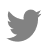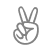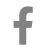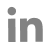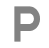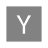# Data Analytics Glossary

Try: Cardinality(SQL), Raw Data, No SQL...

## Central Tendency

#### What is Central Tendency?

Central Tendency is a term used in statistics and databases, it describes the central position of data within a dataset. The central tendency can be referred to as a central location, it is actually the mean value (average) of values in a dataset. Mean is the major measuring term in central tendency where median and more are also used.

#### How does Central Tendency work?

The mean is the most used valid measurement method in central tendency. It is performed by taking the sum of all values in a dataset and dividing it by the number of values in that dataset. The median is the middle value in a magnitude-wise arranged dataset. The mode is the value appearing most of the time in a dataset.

#### Types and Examples:

If a dataset has 5 values, 11, 22, 33, 44, 55. The mean would be taken by Mean= (11 + 22 + 33 + 44 + 55)/5. To find Median, if the values are 22, 33, 44, 11, 55; we'll arrange these values by magnitude like 11, 22, 33, 44, 55. The middle value 33 is the median. The mode is the most appearing value in a dataset.